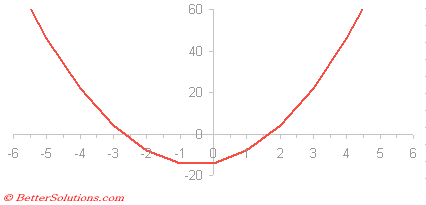Lets suppose we have a quadradic equation and we want to find the roots.
One way to find the roots is to use the Goal Seek.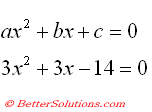### Create a simple Table

You must first create a simple worksheet that contains the following information.
This table can be used to solve any quadratic equation using Goal Seek
Create the following table and the following named ranges:
"C3" = Avalue
"C4" = Bvalue
"C5" = Cvalue
"C7" = Xvalue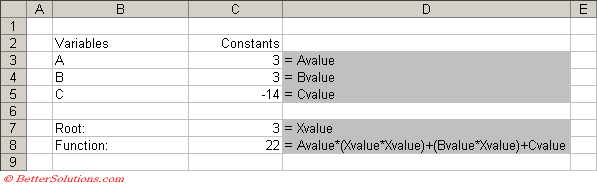### Using Goal Seek

Goal Seeks works by finding the closest result to the starting value (or initial guess).
The problem with using Goal seek is that you have to provide an initial guess.
In this example we are using the initial guess of 3.
Select cell "C8" and select (Tools > Goal Seek).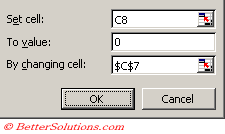if a solution can be found to the formula then the status dialog box is displayed showing the result.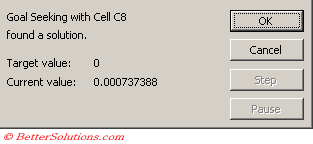Press OK to accept the solution and to change the cell "C7" with the solution found
Pressing Cancel will return cell "C7" to its original guess of 3.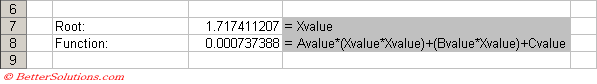This has only found one of the roots though so you will need to repeat the process with another guess.
For our second guess we will use the value -3.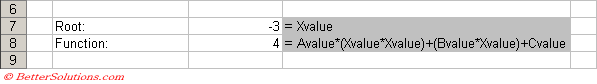Select cell "C7" and select (Tools > Goal Seek)

This time the solution -2.717 has been found which must be other root.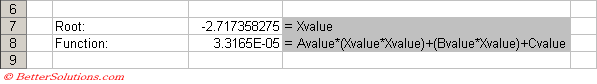It is also possible to find the solutions to a quadratic equation using the following quadratic formula: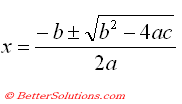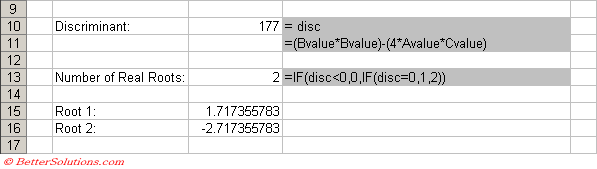### Checking the Results

We can easily check these results by plotting the graph of this function.
The two roots are roughly about -2.7 and 1.7 which correspond with the above results..